2022: SklogWiki celebrates 15 years on-line

# Ideal gas partition function

The canonical ensemble partition function, Q, for a system of N identical particles each of mass m is given by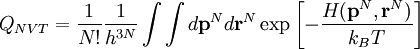$Q_{NVT}=\frac{1}{N!}\frac{1}{h^{3N}}\int\int d{\mathbf p}^N d{\mathbf r}^N \exp \left[ - \frac{H({\mathbf p}^N,{\mathbf r}^N)}{k_B T}\right]$

where h is Planck's constant, T is the temperature and$k_B$ is the Boltzmann constant. When the particles are distinguishable then the factor N! disappears.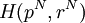$H(p^N, r^N)$ is the Hamiltonian corresponding to the total energy of the system. H is a function of the 3N positions and 3N momenta of the particles in the system. The Hamiltonian can be written as the sum of the kinetic and the potential energies of the system as follows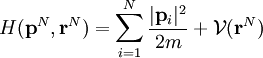$H({\mathbf p}^N, {\mathbf r}^N)= \sum_{i=1}^N \frac{|{\mathbf p}_i |^2}{2m} + {\mathcal V}({\mathbf r}^N)$

Thus we have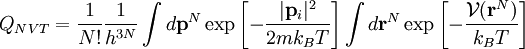$Q_{NVT}=\frac{1}{N!}\frac{1}{h^{3N}}\int d{\mathbf p}^N \exp \left[ - \frac{|{\mathbf p}_i |^2}{2mk_B T}\right] \int d{\mathbf r}^N \exp \left[ - \frac{{\mathcal V}({\mathbf r}^N)} {k_B T}\right]$

This separation is only possible if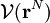${\mathcal V}({\mathbf r}^N)$ is independent of velocity (as is generally the case). The momentum integral can be solved analytically: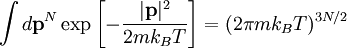$\int d{\mathbf p}^N \exp \left[ - \frac{|{\mathbf p} |^2}{2mk_B T}\right]=(2 \pi m k_B T)^{3N/2}$

Thus we have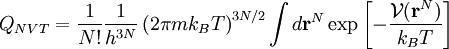$Q_{NVT}=\frac{1}{N!} \frac{1}{h^{3N}} \left( 2 \pi m k_B T\right)^{3N/2} \int d{\mathbf r}^N \exp \left[ - \frac{{\mathcal V}({\mathbf r}^N)} {k_B T}\right]$

The integral over positions is known as the configuration integral,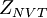$Z_{NVT}$ (from the German Zustandssumme meaning "sum over states")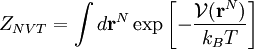$Z_{NVT}= \int d{\mathbf r}^N \exp \left[ - \frac{{\mathcal V}({\mathbf r}^N)} {k_B T}\right]$

In an ideal gas there are no interactions between particles so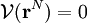${\mathcal V}({\mathbf r}^N)=0$. Thus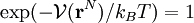$\exp(-{\mathcal V}({\mathbf r}^N)/k_B T)=1$ for every gas particle. The integral of 1 over the coordinates of each atom is equal to the volume so for N particles the configuration integral is given by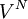$V^N$ where V is the volume. Thus we have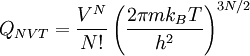$Q_{NVT}=\frac{V^N}{N!}\left( \frac{2 \pi m k_B T}{h^2}\right)^{3N/2}$

If we define the de Broglie thermal wavelength as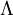$\Lambda$ where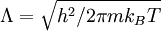$\Lambda = \sqrt{h^2 / 2 \pi m k_B T}$

one arrives at (Eq. 4-12 in )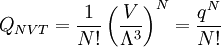$Q_{NVT}=\frac{1}{N!} \left( \frac{V}{\Lambda^{3}}\right)^N = \frac{q^N}{N!}$

where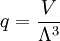$q= \frac{V}{\Lambda^{3}}$

is the single particle translational partition function.

Thus one can now write the partition function for a real system can be built up from the contribution of the ideal system (the momenta) and a contribution due to particle interactions, i.e.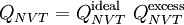$Q_{NVT}=Q_{NVT}^{\rm ideal} ~Q_{NVT}^{\rm excess}$